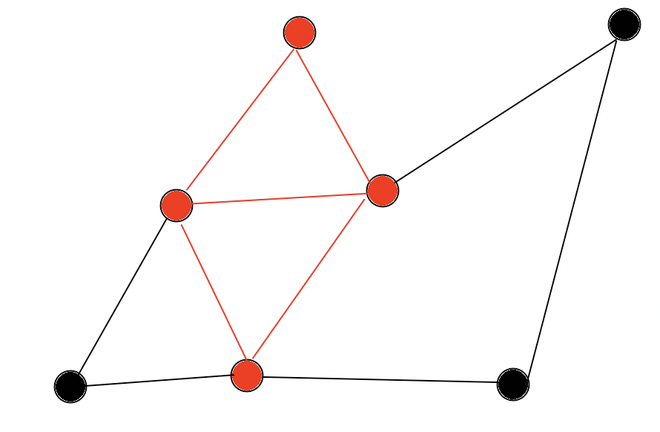# Prove that Dense Subgraph is NP Complete by Generalisation

• Last Updated : 13 Jul, 2022

Prerequisites: NP-Completeness, NP Class, Dense Subgraph

Problem: Given graph G = (V, E) and two integers a and b. A set of a number of vertices of G such that there are at least b edges between them is known as the Dense Subgraph of graph G.

Explanation: To prove the Dense Subgraph problem as NP-completeness by generalization, we are going to prove that it is a generalization of the known NP-complete problem. In this case, we are going to take Clique as the known problem which is already known to be NP-complete, and explained in Proof of Clique Is an NP-Complete and we need to show the reduction from Clique → Dense Subgraph.

Clique is a subset of vertices of an undirected graph such that every two distinct vertices in the clique are adjacent.

Proof:

1. Input Conversion: We need to convert the input from Clique to the input of the Dense Subgraph.

Clique Input:  An undirected graph G(V, E) and integer k.
Dense Subgraph Input : An undirected graph G'(V, E) and two integers a and b.

We are going to transform the input from Clique for Dense Subgraph such that

1. G’ = G(V, E)
2. a = k
3. b = (k * (k – 1))/2

This conversion is going to take O(1) time so it’s polynomial in nature.

2. Output Conversion: We need to convert the solution from Dense Graph to the solution for the Clique problem.

Solution of Dense Graph will result in a set a which would be a Clique of size k as k = a. So direct output from Dense Graph can be used by Clique. Since no conversion is required so it’s again polynomial in nature.

3. Correctness: We have restricted the range of input value b such that (k¦2) with value as (k * (k – 1))/2

Now we are looking for a subgraph having k vertices and are connected by at least (k * (k – 1))/2 edges.

• Since in a complete graph, n vertices can have at most (n * (n – 1))/2 edges between them so we can say that we need to find a subgraph of k vertices that have exactly (k * (k – 1))/2 edges which means output graph should have an edge between each pair of vertices which is nothing but Clique of k vertices.
• Similarly, a Clique of k vertices on a graph G(V, E) must have (k * (k – 1))/2 edges which is nothing but the Dense-Subgraph of graph G(V, E)Red Edges and Vertices denotes a Dense-Subgraph with a = 4 and b = 5

So, this means Dense-Subgraph has a solution↔ Clique has a solution.
The complete reduction takes polynomial time and Clique is NP complete so Dense Subgraph is also NP complete.

Conclusion:

Hence we can conclude that Dense-Subgraph is NP Complete